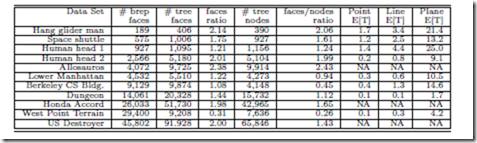# Binary Space Partitioning Trees:BSP Tree as a Hierarchy of Regions.

###### BSP Tree as a Hierarchy of Regions

Another way to look at BSP Trees is to focus on the hierarchy of regions created by the recursive partitioning, instead of focusing on the hyperplanes themselves. This view helps us to see more easily how intersections are eﬃciently computed. The key idea is to think of a BSP Tree region as serving as a bounding volume: each node v corresponds to a convex volume that completely contains all the geometry represented by the sub tree rooted at v. Therefore, if some other geometric entity, such as a point, ray, object, etc., is found to not intersect the bounding volume, then no intersection computations need be performed with any geometry within that volume.

Consider as an example a situation in which we are given some test point and we want to ﬁnd which object if any this point lies in. Initially, we know only that the point lies somewhere space (Figure 20.12).

By comparing the location of the point with respect to the ﬁrst partitioning hyper plane, we can ﬁnd in which of the two regions (a.k.a. bounding volumes) the point lies. This eliminates half of the objects (Figure 20.13).

By continuing this process recursively, we are in eﬀect using the regions as a hierarchy of bounding volumes, each bounding volume being a rough approximation of the geometry it bounds, to quickly narrow our search (Figure 20.14).

For a BSP Tree of a single object, this region-based (volumetric) view reveals how BSP Trees can provide a multi-resolution representation. As one descends a path of the tree, the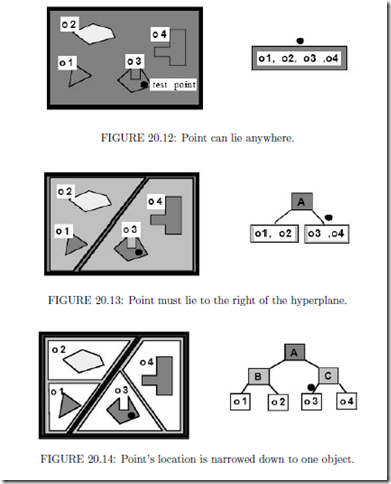regions decrease in size monotonically. For curved objects, the egions converge in the limit to the curve/surface. Truncating the tree produces an approximation, ala the Taylor series of approximations for functions (Figure 20.15).

Tree Merging

The spatial relations between two objects, each represented by a separate tree, can be determined eﬃciently by merging two trees. This is a fundamental operation that can be used to solve a number of geometric problems. These include set operations for CSG modeling as well as collision detection for dynamics. For rendering, merging all object-trees into a single model-tree determines inter-object visibility orderings; and the model-tree can be intersected with the view-volume to eﬃciently cull away oﬀ-screen portions of the scene and provide solid cutaways with the near clipping plane. In the case where objects are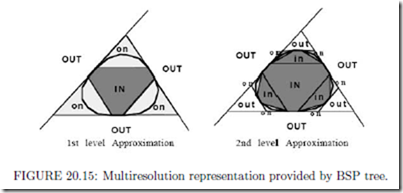both transparent and interpenetrate, tree merging acts as a view independent geometric sorting of the object faces; each tree is used in a manner analogous to the way Merge Sort merges previously sorted lists to quickly created a new sorted list (in our case, a new tree). The model-tree can be rendered using ray tracing, radiosity, or polygon drawing using a far-to-near ordering with alpha blending for transparency. An even better alternative is multi-resolution beam-tracing, since entire occluded subtrees can be eliminated without visiting the contents of the subtree, and distance subtrees can be pruned to the desired resolution. Beam tracing can also be used to eﬃciently compute shadows.

All of this requires as a basic operation an algorithm for merging two trees. Tree merging is a recursive process, which proceeds down the trees in a multi-resolution fashion, going from low-res to high-res. It is easiest to understand in terms of merging a hierarchy of bounding volumes. As the process proceeds, pairs of tree regions, a.k.a. convex bounding volumes, one from each tree, are compared to determine whether they intersect or not. If they do not, the contents of the corresponding subtrees are never compared. This has the eﬀect of “zooming in” on those regions of space where the surfaces of the two objects intersect (Figure 20.16).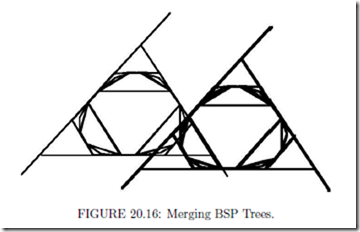The algorithm for tree merging is quite simple once you have a routine for partitioning a tree by a hyper plane into two trees. The process can be thought of in terms of inserting

one tree into the other in a recursive manner. Given trees T1 and T2, at each node of T1 the hyper plane at that node is used to partition T2 into two “halves”. Then each half is merged with the subtree of T1 lying on the same side of the hyper plane. (In actuality, the algorithm is symmetric w.r.t. the role of T1 and T2 so that at each recursive call, T1 can split T2 or T2 can split T1.)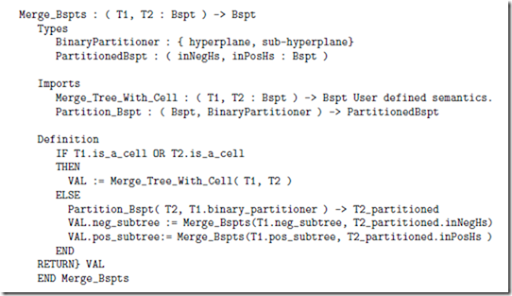While tree merging is easiest to understand in terms of comparing bounding volumes, the actual mechanism uses sub-hyper planes, which is more eﬃcient. A sub-hyper plane is created whenever a region is partitioned by a hyper plane, and it is just the subset of the hyper plane lying within that region. In fact, all of the illustrations of trees we have used are drawings of sub-hyper planes. In 3D, these are convex polygons, and they separate the two child regions of an internal node. Tree merging uses sub-hyper planes to simultaneously determine the spatial relations of four regions, two from each tree, by comparing the two sub-hyper planes at the root of each tree. For 3D, this is computed using two applications of convex-polygon clipping to a plane, and there are three possible outcomes: intersecting, non-intersecting and coincident (Figure 20.17). This is the only overtly geometric computation in tree merging; everything else is data structure manipulation.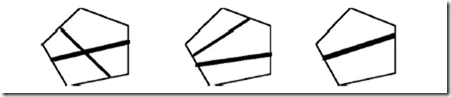FIGURE 20.17: Three cases (intersecting, non-intersecting, coincident) when comparing sub-hyper planes during tree merging.

Good BSP Trees

For any given set, there exist an arbitrary number of diﬀerent BSP Trees that can represent that set. This is analogous to there being many diﬀerent programs for computing the same function, since a BSP Tree may in fact be interpreted as a computation graph specifying a particular search of space. Similarly, not all programs/algorithms are equally eﬃcient, and not all searches/trees are equally eﬃcient. Thus the question arises as to what constitutes a good BSP Tree. The answer is a tree that represents the set as a sequence of approximations. This provides a multi-resolution representation. By pruning the tree at various depths, diﬀerent approximations of the set can be created. Each pruned subtree is replaced with a cell containing a low degree polynomial approximation of the set represented by the subtree (Figures 20.18 and 20.19).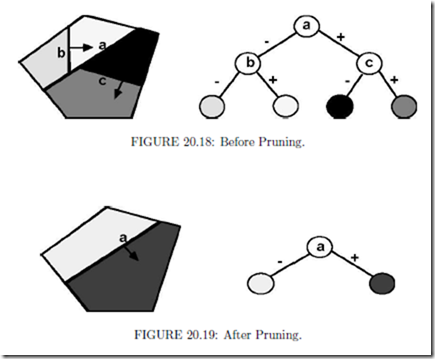In Figure 20.20, we show two quite diﬀerent ways to represent a convex polygon, only the second of which employs the sequence of approximations idea. The tree on the left subdivides space using lines radiating from the polygonal center, splitting the number of faces in half at each step of the recursive subdivision. The hyperplanes containing the polygonal edges are chosen only when the number of faces equals one, and so are last along any path. If the number of polygonal edges is n, then the tree is of size O(n) and of depth O(log n). In contrast, the tree on the right uses the idea of a sequence of approximations. The ﬁrst three partitioning hyperplanes form a ﬁrst approximation to the exterior while the next three form a ﬁrst approximation to the interior. This divides the set of edges into three sets. For each of these, we choose the hyperplane of the middle face by which to partition, and by doing so reﬁne our representation of the exterior. Two additional hyperplanes reﬁne the interior and divide the remaining set of edges into two nearly equal sized sets. This process precedes recursively until all edges are in partitioning hyper planes. Now, this tree is also of size O(n) and depth O(log n), and thus the worst case, say for point classiﬁcation, is the same for both trees. Yet they appear to be quite diﬀerent.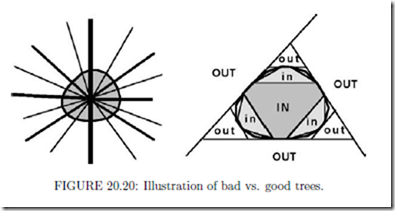This apparent qualitative diﬀerence can be made quantitative by, for example, considering the expected case for point classiﬁcation. With the ﬁrst tree, all cells are at depth log n, so the expected case is the same as the worst case regardless of the sample space from which a point is chosen. However, with the second tree, the top three out-cells would typically constitute most of the sample space, and so a point would often be classiﬁed as OUT by, on average, two point-hyperplane tests. Thus the expected case would converge to O(1) as the ratio of polygon-area/sample-area approaches 0. For line classiﬁcation, the two trees diﬀer not only in the expected case but also in the worst case: O(n) vs. O(log n). For merging two trees the diﬀerence is O(n2) vs. O(n log n). This reduces even further to O(log n) when the objects are only contacting each other, rather overlapping, as is the case for collision detection. However, there are worst case “basket weaving” examples that do require O(n2) operations. These are geometric versions of the Cartesian product, as for example when a checkerboard is constructed from n horizontal strips and n vertical strips to produce n × n squares. These examples, however, violate the Principle of Locality: that geometric features are local not global features. For almost all geometric models of physical objects, the geometric features are local features. Spatial partitioning schemes can accelerate computations only when the features are in fact local, otherwise there is no signiﬁcant subset of space that can be eliminated from consideration. The key to a quantitative evaluation, and also generation, of BSP Trees is to use expected case models, instead of worst-case analysis. Good trees are ones that have low expected cost for the operations and distributions of input of interest. This means, roughly, that high probability regions can be reached with low cost, i.e. they have short paths from the root to the corresponding node, and similarly low probability regions should have longer paths.

This is exactly the same idea used in Huﬀman codes. For geometric computation, the probability of some geometric entity, such as a point, line segment, plane, etc., lying in some arbitrary region is typically correlated positively to the size of the region: the larger the region the greater the probability that a randomly chosen geometric entity will intersect that region. To compute the expected cost of a particular operation for a given tree, we need to know at each branch in the tree the probability of taking the left branch, p, and the probability of taking the right branch p+. If we assign a unit cost to the partitioning operation, then we can compute the expected cost exactly, given the branch probabilities, using the following recurrence relation: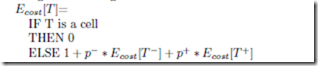This formula does not directly express any dependency upon a particular operation; those characteristics are encoded in the two probabilities pand p+. Once a model for these is speciﬁed, the expected cost for a particular operation can be computed for any tree.

As an example, consider point classiﬁcation in which a random point is chosen from a uniform distribution over some initial region R. For a tree region of r with child regions r+ and r, we need the conditional probability of the point lying in r+ and r, given that it lies in r. For a uniform distribution, this is determined by the sizes of the two child-regions relative to their parent: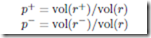Similar models have been developed for line, ray and plane classiﬁcation. Below we describe how to use these to build good trees.

Converting B-reps to Trees

Since humans do not see physical objects in terms of binary trees, it is important to know how such a tree be constructed from something that is more intuitive. The most common method is to convert a boundary representation, which corresponds more closely to how humans see the world, into a tree. In order for a BSP Tree to represent a solid object, each cell of the tree must be classiﬁed as being either entirely inside or outside of the object; thus, each leaf node corresponds to either an in-cell or an out-cell. The boundary of the set then lies between in-cells and out-cells; and since the cells are bounded by the partitioning hyper planes, it is necessary for the entire boundary to lie in the partitioning hyperplanes (Figure 20.21).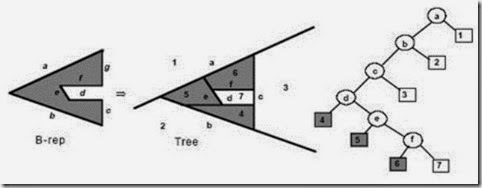FIGURE 20.21: B-rep and Tree representation of a polygon.

Therefore, we can convert from a b-rep to a tree simply by using all of the face hyper planes as partitioning hyper planes. The face hyper planes can be chosen in any order and the

resulting tree will always generate a convex decomposition of the interior and the exterior. If the hyper plane normals of the b-rep faces are consistently oriented to point to the exterior, then all left leaves will be in-cells and all right leaves will be out-cells. The following algorithm summarizes the process.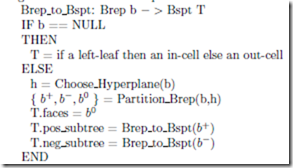However, this does not tell us in what order to choose the hyper planes so as to produce the best trees. Since the only known method for ﬁnding the optimal tree is by exhaustive enumeration, and there are at least n! trees given n unique face hyper planes, we must employ heuristics. In 3D, we use both the face planes as candidate partitioning hyper planes, as well as planes that go through face vertices and have predetermined directions, such as aligned with the coordinates axes. Given any candidate hyper plane, we can try to predict how eﬀective it will be using expected case models; that is, we can estimate the expected cost of a sub tree should we choose this candidate to be at its root. We will then choose the least cost candidate. Given a region r containing boundary b which we are going to partition by a candidate h, we can compute exactly p+ and pfor a given operation, as well as the size of b+ and b. However, we can only estimate E cost[T +] and Ecost[T ]. The estimators for these values can depend only upon a few simple properties such as number of faces in each half space, how many faces would be split by this hyper plane, and how many faces lie on the hyperplane (or area of such faces). Currently, we use |b+ n for Ecost[T +], where n typically varies between .8 and .95, and similarly for Ecost[T ]. We also include a small penalty for splitting a face by increasing its contribution to b+ and bfrom 1.0 to somewhere between 1.25 and 1.75, depending upon the object. We also favor candidates containing larger surface area, both in our heuristic evaluation and by ﬁrst sorting the faces by surface area and considering only the planes of the top k faces as candidates. One interesting consequence of using expected case models is that choosing the candidate that attempts to balance the tree is usually not the best; instead the model prefers candidates that place small amounts of geometry in large regions, since this will result in high probability and low cost subtrees, and similarly large amounts of geometry in small regions. Balanced is optimal only when the geometry is uniformly distributed, which is rarely the case (Figure 20.22). More importantly, minimizing expected costs produces trees that represents the object as a sequence of approximations, and so in a multi-resolution fashion.

Boundary Representations vs. BSP Trees

Boundary Representations and BSP Trees may be viewed as complementary representations expressing diﬀerence aspects of geometry, the former being topological, the later expressing hierarchical set membership. B-reps are well suited for interactive speciﬁcation of geometry, expressing topological deformations, and scan-conversion. BSP Trees are well suited for intersection and visibility calculations. Their relationship is probably more akin to the capacitor vs. inductor, than the tube vs. transistor.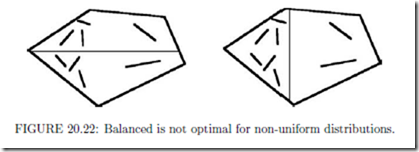The most often asked question is what is the size of a BSP Tree representation of a polyhedron vs. the size of its boundary representation. This, of course, ignores the fact that expected cost, measured over the suite of operations for which the representation will be used, is the appropriate metric. Also, boundary representations must be supplemented by other devices, such as octrees, bounding volumes hierarchies, and z-buﬀers, in order to achieve an eﬃcient system; and so the cost of creating and maintaining these structure should be brought into the equation. However, given the intrinsic methodological diﬃculties in per- forming a compelling empirical comparison, we will close with a few examples giving the original number of b-rep faces and the resulting tree using our currently implemented tree construction machinery. The ﬁrst ratio is number-of-tree-faces/number-of-brep-faces. The second ratio is number-of-tree-nodes/number-of-brep-faces, where number-of-tree-nodes is the number of internal nodes. The last column is the expected cost in terms of point, line and plane classiﬁcation, respectively, in percentage of the total number of internal nodes, and where the sample space was a bounding box 1.1 times the minimum axis-aligned bounding box. These numbers are pessimistic since typical sample spaces would be much larger than an object’s bounding box. Also, the heuristics are controlled by 4 parameters, and these numbers were generated, with some exceptions, without a search of the parameter space but rather using default parameters. There are also quite a number of ways to improve the conversion process, so it should be possible to do even better.